# What is Sensitivity Analysis?

A guide to sensitivity analysis

## What is Sensitivity Analysis?

Sensitivity Analysis is a tool used in financial modeling to analyze how the different values of a set of independent variables affect a specific dependent variable under certain specific conditions. In general, Sensitivity Analysis is used in a wide range of fields, ranging from biology and geography to economics and engineering.

It is especially useful in the study and analysis of a “Black Box Processes” where the output is an opaque function of several inputs. An opaque function or process is one which for some reason can’t be studied and analyzed. For example, climate models in geography are usually very complex. As a result, the exact relationship between the inputs and outputs are not well understood.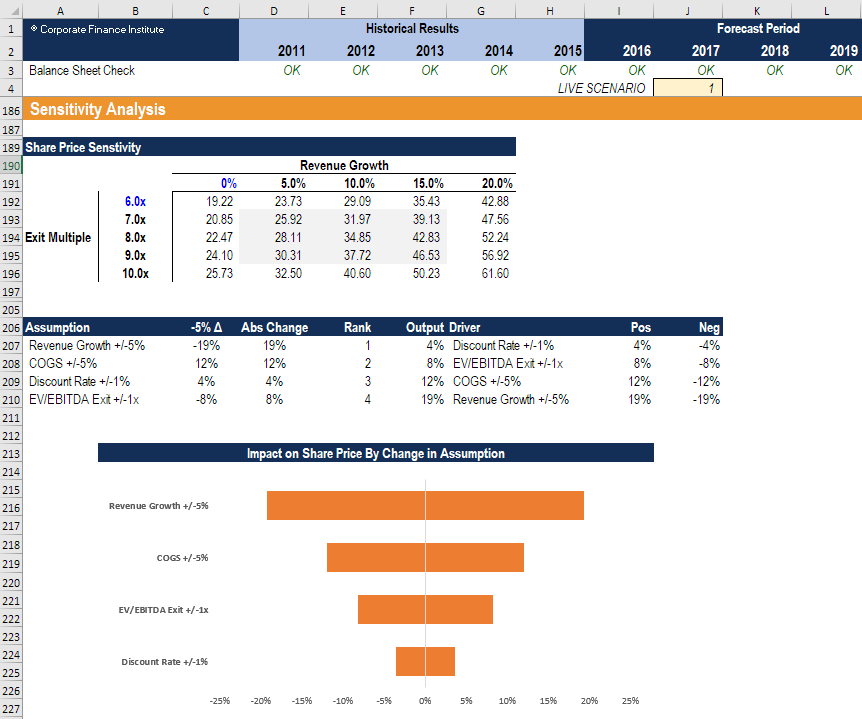Image from CFI’s Scenario & Sensitivity Analysis in Excel Course

### What-If Analysis

A Financial Sensitivity Analysis, also known as a What-If analysis or a What-If simulation exercise, is most commonly used by financial analysts to predict the outcome of a specific action when performed under certain conditions.

Financial Sensitivity Analysis is done within defined boundaries that are determined by the set of independent (input) variables.

For example, Sensitivity Analysis can be used to study the effect of a change in interest rates on bond prices if the interest rates increased by 1%.  The “What-If” question would be: “What would happen to the price of a bond If interest rates went up by 1%?”. This question is answered with sensitivity analysis.

The analysis is performed in Excel under the Data section of the ribbon and the “What-if Analysis” button, which contains Goal Seek and Data Table.  These are both taught step by step in our free Excel Crash Course.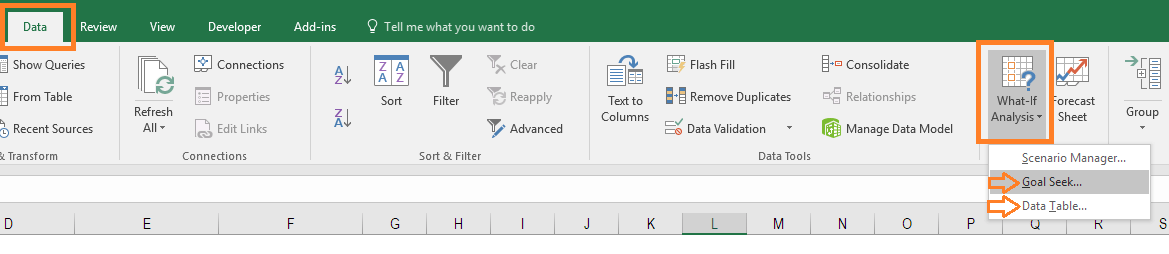### Sensitivity Analysis Example

John is in charge of sales for HOLIDAY CO that sells Christmas decorations at a shopping mall. John knows that the holiday season is approaching and that the mall will be crowded. He wants to find out whether an increase in customer traffic at the mall will raise the total sales revenue of HOLIDAY CO and if so, by how much.

The average price of a packet of Christmas decorations is \$20 and during the previous year’s holiday season, HOLIDAY CO sold 500 packs of Christmas decorations, resulting in total sales worth \$10,000.

After carrying out a Financial Sensitivity Analysis, John determines that a 10% increase in customer traffic at the mall results in a 7% increase in the number of sales.

Using this information, John can predict how much money company XYZ will generate if customer traffic increases by 20%, 40%, or 100%.

Based on John’s Financial Sensitivity Analysis, these will result in an increase in revenue by 14%, 28%, and 70%, respectively.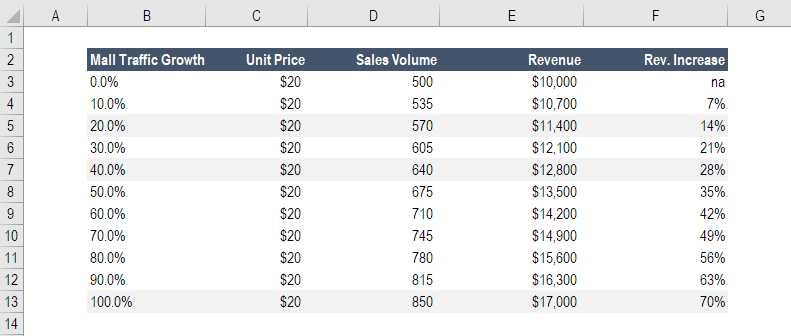Learn how to build a table like this in our Free Excel Crash Course!

### Sensitivity Analysis vs. Scenario Analysis

It is important not to confuse Financial Sensitivity Analysis with Financial Scenario Analysis. Although similar to some degree, the two have some key differences.

Sensitivity Analysis is used to understand the effect of a set of independent variables on some dependent variable under certain specific conditions. For example, a financial analyst wants to find out the effect of a company’s net working capital on its profit margin. The analysis will involve all the variables that have an impact on the company’s profit margin such as the cost of goods sold, workers’ wages and managers’ wages, etc. The analysis will isolate each of these fixed and variable costs and record all the possible outcomes.

Scenario Analysis, on the other hand, would require the financial analyst to describe a specific scenario in detail. Scenario Analysis is usually done to analyze situations involving major shocks such as a global market shift or a major change in the business.

After specifying the details of the scenario, the analyst would then have to specify all the variables, so that they align with the scenario. The result is a very comprehensive picture of the future (a discrete scenario). The analyst would know the full range of outcomes, given all the extremes, and would have an understanding of what the outcomes would be, given a specific set of variables defined by a specific real-life scenario.

### Advantages of Financial Sensitivity Analysis

There are many important reasons to perform sensitivity analysis:

• Sensitivity Analysis adds credibility to any type of financial model by testing the model across a wide set of possibilities.
• Financial Sensitivity Analysis allows the analyst to be flexible with the boundaries within which to test the sensitivity of the dependent variables to the independent variables. For example, the model to study the effect of a 5-point change in interest rates on bond prices would be different from the financial model that would be used to study the effect of a 20-point change in interest rates on bond prices.
• Sensitivity analysis helps one make informed choices. Decision-makers use the model to understand how responsive the output is to changes in certain variables. This relationship can help an analyst in deriving tangible conclusions and be instrumental in making optimal decisions.

### Best Practices in Sensitivity Analysis

#### #1 Layout in Excel

Layout, structure, and planning are all important for good sensitivity analysis in Excel.  If a model is not well organized both the creator and users of the model will be confused and the analysis will be prone to error.

The most important points to keep in mind for layout in Excel include:

• Place all assumptions in one area of the model
• Format all assumptions/inputs in a unique font color so they are easy to identify
• Think carefully about what to test – only the most important assumptions
• Understand the relationship (correlation) between dependent and independent variables (linear, nonlinear?)
• Create charts and graphs that allow users to visualize the data
• Create a separate area for the analysis using grouping (see example below)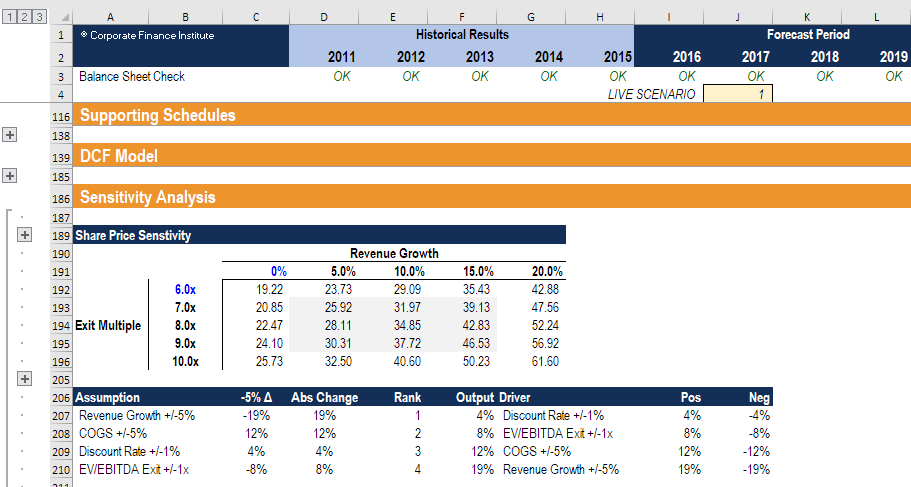#### #2 Direct versus indirect methods

The direct method involves substituting different numbers into an assumption in a model.

For example, if the revenue growth assumption in a model is 10% year-over-year (YoY), then the revenue formula is = (last year revenue) x (1 + 10%). In the direct approach, we substitute different numbers to replace the growth rate, like 0%, 5%, 15%, and 20% and see what the resulting revenue dollars are.

The indirect method (as shown below) inserts a percent change into formulas in the model, instead of directly changing the value of an assumption.

With the same example as above, if the revenue growth assumption in a model is 10% year-over-year (YoY), then the revenue formula is = (last year revenue) x (1 + 10%).  Instead of changing 10% to some other number, we can change the formula to be = (last year revenue) x (1 + (10% + X)). Where X is a value contained down in the sensitivity analysis area of the model.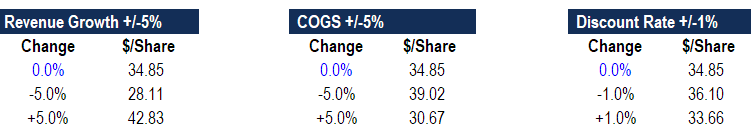Learn how to do this step by step and launch our sensitivity analysis course now!

#### #3 Tables, charts, and graphs

Sensitivity analysis can be challenging to comprehend even by the most informed and technically savvy finance professionals, so it’s important to be able to express the results in a manner that’s easy to follow.

Data tables are a great way of showing the impact on a dependent variable by changing up to two independent variables.  Below is an example of a data table that clearly shows the impact of changes in revenue growth and EV/EBITDA multiple on a company’s share price.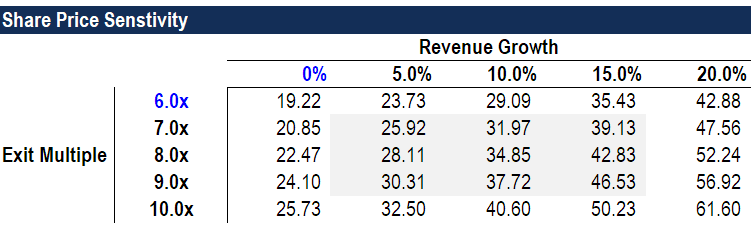Tornado Charts can be a great way of showing the impact of changes to many variables at once.  They are called Tornado Charts because they are sorted from the most impactful to least impactful in a way that shapes the chart like a tornado cone.  To learn how to build these charts, launch our sensitivity analysis in Excel course now!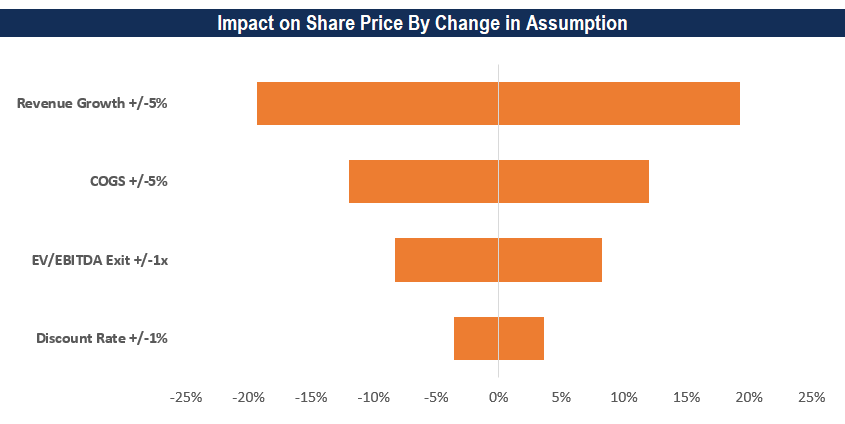### Video Explanation of Sensitivity Analysis

Watch this short video to quickly understand the main concepts covered in this guide, including Direct and Indirect methods.

### Related articles and guides

Thank you for reading this guide to sensitivity analysis. CFI is the official global provider of the Financial Modeling and Valuation Analyst (FMVA) designation, a leading credential for financial analysts. To learn more about financial modeling, these free resources will be helpful:

• Scenario Analysis
• Analysis of Financial Statements
• DCF Modeling Guide
• Financial Modeling Best Practices

### Financial Modeling Certification

Become a certified Financial Modeling and Valuation Analyst (FMVA)® by completing CFI’s online financial modeling classes!